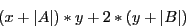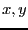Next: Solving systems of distance Up: Solving with Interval Analysis Previous: The GradientNonLinear procedure   Contents

Solving systems with determinants

In some cases systems may involve determinants: for example in algebraic geometry the resultant of two algebraic equations is defined as the determinant of the Sylvester matrix. To get an analytical form of the equations it is therefore necessary to compute the determinant: but in some cases this may be quite difficult as the expression may be quite large, even for sparse matrix. A mechanism in ALIAS enable to get the interval evaluation of an equation which include determinant without having to provide the analytical form of the determinant but only the interval evaluation of the components of the matrix. This mechanism is based on procedures that compute the interval evaluation of the determinant of an interval-valued matrix (see section 7.1).

Using these procedures it is possible to design the equation evaluation procedure that are used in the general solving procedure of ALIAS as described in 2.3.4.3. Assume for example that you have to evaluate the expressionwhere A, B are two matrices of dimension 6. Assume that V is an interval vector which contain the interval values forand that you have to return the interval evaluation of this equation in a interval vector W. Then you may write the following procedure:
INTERVAL_MATRIX A(6,6),B(6,6);
A=Compute_A(V) //compute A for the interval value of x,y
B=Compute_B(V) //compute B for the interval value of x,y
W(1)=(V(1)+Medium_Determinant(A))*V(2)+2*(V(2)+Medium_Determinant(B));
You must be however careful when using this procedure in a denominator as the presence of 0 in the interval evaluation of the determinant is not checked, which will lead to an error when computing the interval evaluation of an equation (see section 2.1.1.3).

Note that the MakeF procedure of the ALIAS-Maple package is able to produce efficient code for an equation file even if unexpanded determinants are present in the equation.

There are also procedures to compute the derivatives of a determinant Note that the MakeJ procedure of the ALIAS-Maple package is able to produce a procedure compatible with the requirements of the gradient procedure required by the library (see section 2.4.2.2) even if determinants are present in the equation.

There are also procedures to compute the second order derivatives of a determinant Note that the MakeH procedure of the ALIAS-Maple package is able to produce a procedure compatible with the requirements of the hessian procedure required by the library (see section 2.5.2.1) even if determinants are present in the equation.Next: Solving systems of distance Up: Solving with Interval Analysis Previous: The GradientNonLinear procedure   Contents
Jean-Pierre Merlet 2012-12-20